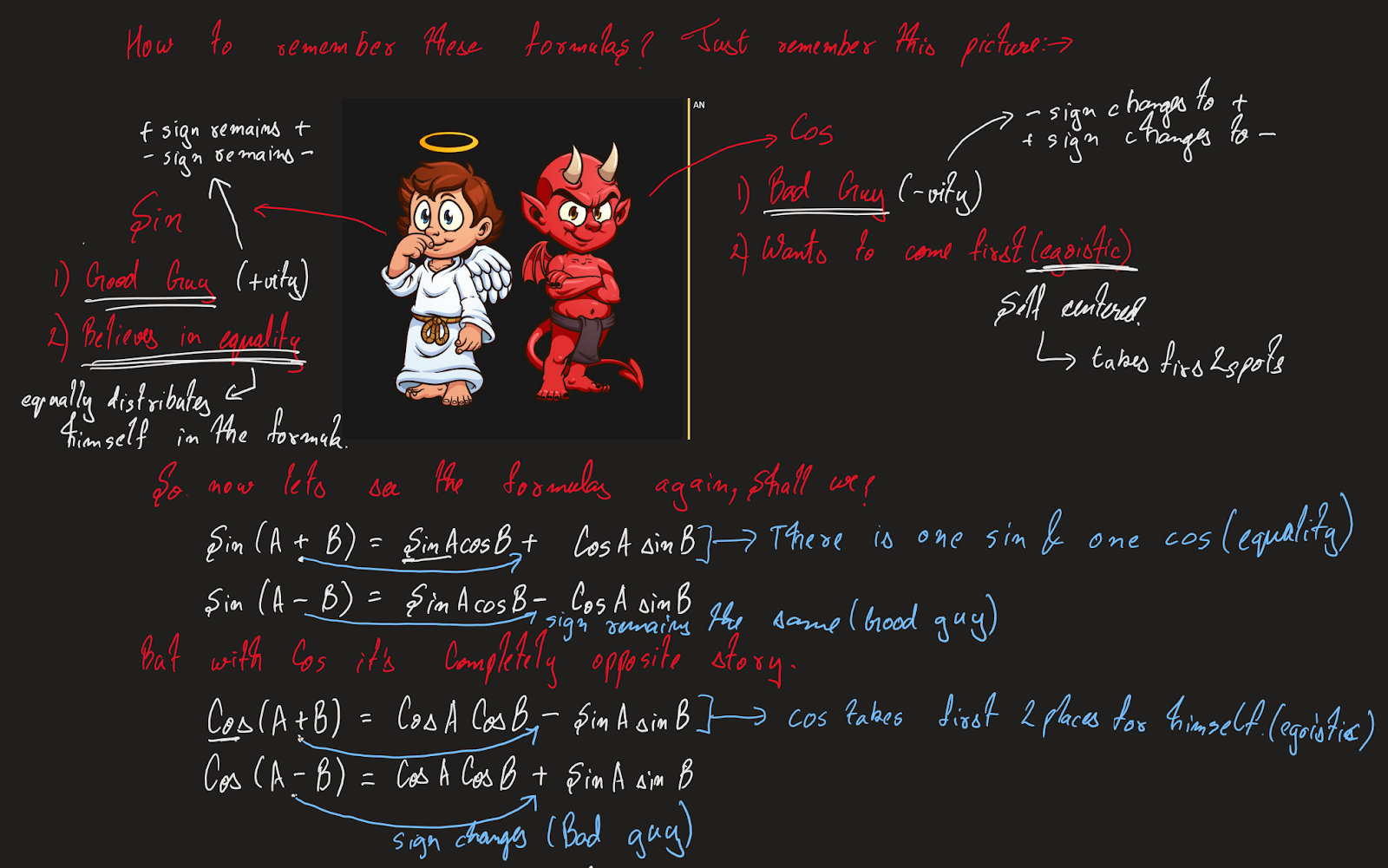# Trigonometry

## Introduction

Simplifying trigonometric expressions can be helpful when we are solving trigonometric equations or proving trigonometric identities. We can use the basic trigonometric ratios, combined and double-angle formulas, as well as reciprocal and other identities to do so.

The following are common formulas and identities we can use as tools to simplify trigonometric expressions:Watch this video for proofs of the Pythagorean Identities:

You can use the information on the following image to remember the combined angle formulas:## Examples

See this video where we review the basic trigonometric ratios and identities that we can use to simplify trigonometric expressions, as well as solutions to the following example:

Example: Simplify the following trigonometric expressions:
 1. $$(sin\theta\cdot tan\theta)(csc\theta+cot\theta)$$ (starts at 1:59) 2. $$\frac{tan(x)\cdot csc^2(x)}{1+tan^2(x)}$$ (starts at 3:35)

See this video where we review the combined angle formulas that we can use to simplify trigonometric expressions, as well as solutions to the following example:

Example: Simplify the following trigonometric expressions:
 1. $$sin3x\cdot cosx-sinx\cdot cos3x$$ (starts at 5:09) 2.$$cos(x+\pi)\cdot cos(x-\pi)+sin(x+\pi)\cdot sin(x-\pi)$$ (starts at 6:03)